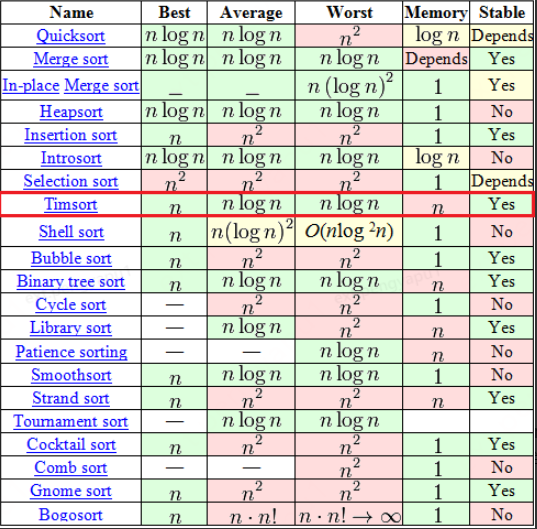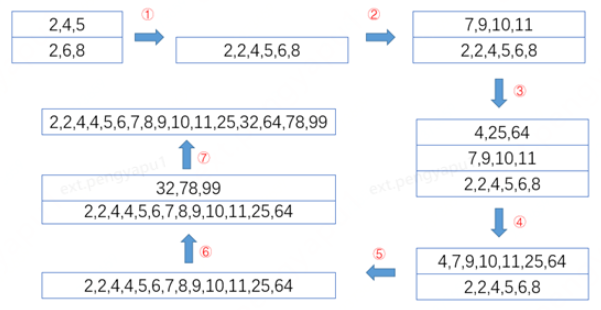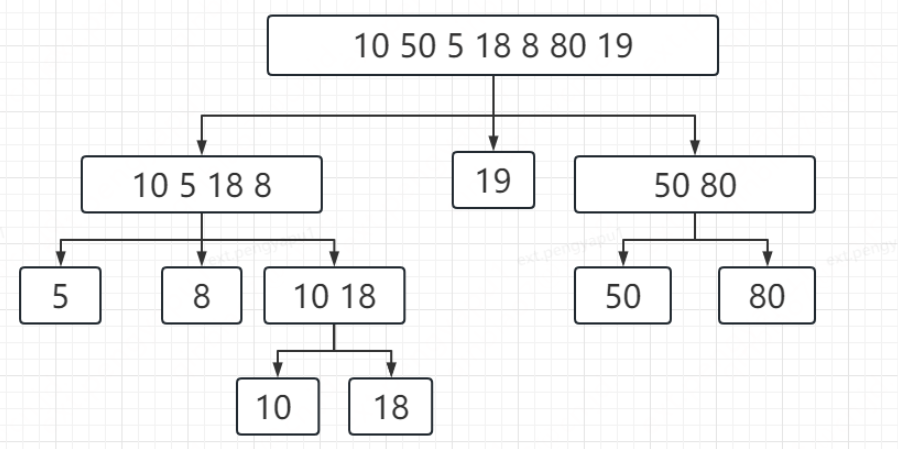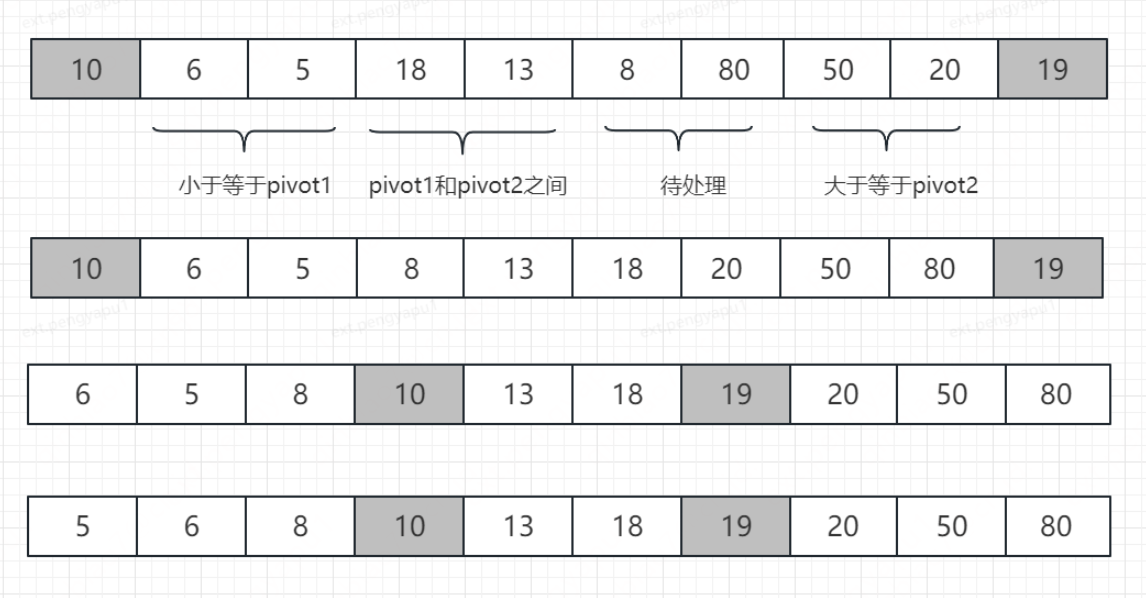# Java 集合中的排序演算法淺析

## 2.  案例

``````List<Integer> list = Arrays.asList(10, 50, 5, 14, 16, 80);
System.out.println(list.stream().sorted().collect(Collectors.toList()));``````

``````@Override
public void end() {
Arrays.sort(array, 0, offset, comparator);
downstream.begin(offset);
if (!cancellationWasRequested) {
for (int i = 0; i < offset; i++)
downstream.accept(array[i]);
}
else {
for (int i = 0; i < offset && !downstream.cancellationRequested(); i++)
downstream.accept(array[i]);
}
downstream.end();
array = null;
}``````

``````List<Integer> list1 = Arrays.asList(10, 50, 5, 14, 16, 80);
System.out.println(list1.stream().sorted().collect(Collectors.toList()));

List<Integer> list2 = Lists.newArrayList();
Collections.sort(list2);
System.out.println(list2);
// 輸出：
// [5, 10, 14, 16, 50, 80]
// [5, 10, 14, 16, 50, 80]``````

## 2.  Collections.sort 方法介紹

Collections類中關於sort方法定義如下：

``````public static <T extends Comparable<? super T>> void sort(List<T> list) {
list.sort(null);
}``````

``````1. Sorts the specified list into ascending order, according to the Comparable natural ordering of its elements.
2. This sort is guaranteed to be stable equal elements will not be reordered as a result of the sort.
3. The specified list must be modifiable, but need not be resizable.``````

``````default void sort(Comparator<? super E> c) {
Object[] a = this.toArray();
Arrays.sort(a, (Comparator) c);
ListIterator<E> i = this.listIterator();
for (Object e : a) {
i.next();
i.set((E) e);
}
}``````

``````* The default implementation obtains an array containing all elements in
* this list, sorts the array, and iterates over this list resetting each
* element from the corresponding position in the array. (This avoids the
* n<sup>2</sup> log(n) performance that would result from attempting
* to sort a linked list in place.)``````

``````public static <T> void sort(T[] a, Comparator<? super T> c) {
if (c == null) {
sort(a);
} else {
if (LegacyMergeSort.userRequested)
legacyMergeSort(a, c);
else
TimSort.sort(a, 0, a.length, c, null, 0, 0);
}
}``````

## 3.  TimSort 演算法介紹

Timsort是一個自適應的、混合的、穩定的排序演算法，是由Tim Peter於2002年發明的，最早應用在Python中，現在廣泛應用於Python、Java、Android 等語言與平臺中，作為基礎的排序演算法使用。其中Java語言的Collection.sort在JDK1.6使用的是普通的歸併排序，歸併排序雖然時間複雜度低，但是空間複雜度要求較高，所以從JDK1.7開始就更改為了TimSort演算法。

Timsort 的時間複雜度是 O(n log n)，與歸併排序的時間複雜度相同，那它的優勢是啥呢，實際上可以認為TimSort排序演算法是歸併排序演算法的優化版，從它的三個特徵就可以看出，第二個特徵“混合的”，沒錯，它不單純是一種演算法，而是融合了歸併演算法和二分插入排序演算法的精髓，因此能夠在排序效能上表現優異。其它兩個特徵自適應和穩定性會在文章後面講到。首先從演算法效能統計上做個對比：### 3.1 TimSort的核心思想

run是指連續升序或者連續降序的最長子序列（降序和升序可以相互轉換），而minrun是一個設定值，實際上是每個run的長度最小值。所以TimSort會對待排序序列進行劃分，找出連續有序的子序列，如果子序列長度不滿足這點要求，就將後續資料插入到前面的子序列中。

（1）x1 <= x2 + x3

（2）x1 <= x2

（1）首先第一個run0是[2,6,8]，而第二個run1是[2,4,5]，此時依次將其放入棧中，發現滿足第二個條件，這兩個run進行合併，合併後將舊序列從棧中彈出，得到新的run0是[2,2,4,5,6,8]，再次壓入棧中。

（2）繼續從原序列中找到新的run1是[7,9,10,11]，壓入棧中，此時run0和run1不滿足條件不需要合併。繼續從原序列中找到run2是[4,25,64]，壓入棧中，此時滿足第一個條件，這裡的run1和run2需要進行合併，合併後將舊序列從棧中彈出，新run1是[4,7,9,10,11,25,64]，壓入棧中。

（3）此時發現run0和run1滿足第二個條件，繼續合併彈出舊序列，得到新run0是[2,2,4,4,5,6,7,8,9,10,11,25,64]，壓入棧中。

（4）繼續從原序列中找到新的run1是[32,78,99]，壓入棧中。此時發現沒有更多元素，而條件是不滿足的，依然進行一次合併，彈出舊序列，壓入合併後的新子序列run0是[2,2,4,4,5,6,7,8,9,10,11,25,32,64,78,99]

（5）此時將run0拷貝到原序列就完成了排序### 3.2 Java原始碼

``````static <T> void sort(T[] a, int lo, int hi, Comparator<? super T> c,
T[] work, int workBase, int workLen) {
assert c != null && a != null && lo >= 0 && lo <= hi && hi <= a.length;

int nRemaining  = hi - lo;
if (nRemaining < 2)
return;

if (nRemaining < MIN_MERGE) {
int initRunLen = countRunAndMakeAscending(a, lo, hi, c);
binarySort(a, lo, hi, lo + initRunLen, c);
return;
}

TimSort<T> ts = new TimSort<>(a, c, work, workBase, workLen);
int minRun = minRunLength(nRemaining);
do {
int runLen = countRunAndMakeAscending(a, lo, hi, c);

if (runLen < minRun) {
int force = nRemaining <= minRun ? nRemaining : minRun;
binarySort(a, lo, lo + force, lo + runLen, c);
runLen = force;
}

ts.pushRun(lo, runLen);
ts.mergeCollapse();

lo += runLen;
nRemaining -= runLen;
} while (nRemaining != 0);

assert lo == hi;
ts.mergeForceCollapse();
assert ts.stackSize == 1;
}``````

• 如果待排序陣列長度為2的n次冪，比如1024，則minRun = 32/2 = 16
• 其它情況的時候，逐位右移，直到找到介於16<=k<=32的值。

``````private static int minRunLength(int n) {
assert n >= 0;
int r = 0;      // Becomes 1 if any 1 bits are shifted off
while (n >= MIN_MERGE) {
r |= (n & 1);
n >>= 1;
}
return n + r;
}``````

（1） 計算最小升序的run長度，如果小於minRun，使用二分插入排序將run的長度補充到minRun要求的長度。

（2） ts.pushRun(lo, runLen) ，通過棧記錄每個run的長度，這裡lo是run的第一個元素的索引用來標記操作的是哪個run，runLen是run的長度。

``````private void pushRun(int runBase, int runLen) {
this.runBase[stackSize] = runBase;
this.runLen[stackSize] = runLen;
stackSize++;
}``````

（3）ts.mergeCollapse();  通過計算前面提到的兩個run合併的限定條件，分別是：

• runLen[n-1] <= runLen[n] + runLen[n+1]
• runLen[n] <= runLen[n + 1]
``````private void mergeCollapse() {
while (stackSize > 1) {
int n = stackSize - 2;
if (n > 0 && runLen[n-1] <= runLen[n] + runLen[n+1]) {
if (runLen[n - 1] < runLen[n + 1])
n--;
mergeAt(n);
} else if (runLen[n] <= runLen[n + 1]) {
mergeAt(n);
} else {
break; // Invariant is established
}
}
}``````

（4） 這裡的mergeAt(n) 歸併排序過程，之前有提到是經過優化後所謂gallop模式的歸併排序，具體表現在方法中的gallopRight和gallopLeft方法。

``````int k = gallopRight(a[base2], a, base1, len1, 0, c);
assert k >= 0;
base1 += k;
len1 -= k;
if (len1 == 0)
return;

len2 = gallopLeft(a[base1 + len1 - 1], a, base2, len2, len2 - 1, c);
assert len2 >= 0;
if (len2 == 0)
return;``````

## 4.  DivalQuickSort 演算法介紹

``````public static void sort(int[] a) {
DualPivotQuicksort.sort(a, 0, a.length - 1, null, 0, 0);
}``````

### 4.1 DivalQuickSort 核心思想### 4.2 Java原始碼

``````static void sort(int[] a, int left, int right,
int[] work, int workBase, int workLen) {
if (right - left < QUICKSORT_THRESHOLD) {
sort(a, left, right, true);
return;
}

int[] run = new int[MAX_RUN_COUNT + 1];
int count = 0; run = left;

for (int k = left; k < right; run[count] = k) {
if (a[k] < a[k + 1]) { // ascending
while (++k <= right && a[k - 1] <= a[k]);
} else if (a[k] > a[k + 1]) { // descending
while (++k <= right && a[k - 1] >= a[k]);
for (int lo = run[count] - 1, hi = k; ++lo < --hi; ) {
int t = a[lo]; a[lo] = a[hi]; a[hi] = t;
}
} else { // equal
for (int m = MAX_RUN_LENGTH; ++k <= right && a[k - 1] == a[k]; ) {
if (--m == 0) {
sort(a, left, right, true);
return;
}
}
}

if (++count == MAX_RUN_COUNT) {
sort(a, left, right, true);
return;
}
}

if (run[count] == right++) {
run[++count] = right;
} else if (count == 1) {
return;
}

byte odd = 0;
for (int n = 1; (n <<= 1) < count; odd ^= 1);

int[] b;
int ao, bo;
int blen = right - left;
if (work == null || workLen < blen || workBase + blen > work.length) {
work = new int[blen];
workBase = 0;
}
if (odd == 0) {
System.arraycopy(a, left, work, workBase, blen);
b = a;
bo = 0;
a = work;
ao = workBase - left;
} else {
b = work;
ao = 0;
bo = workBase - left;
}

for (int last; count > 1; count = last) {
for (int k = (last = 0) + 2; k <= count; k += 2) {
int hi = run[k], mi = run[k - 1];
for (int i = run[k - 2], p = i, q = mi; i < hi; ++i) {
if (q >= hi || p < mi && a[p + ao] <= a[q + ao]) {
b[i + bo] = a[p++ + ao];
} else {
b[i + bo] = a[q++ + ao];
}
}
run[++last] = hi;
}
if ((count & 1) != 0) {
for (int i = right, lo = run[count - 1]; --i >= lo;
b[i + bo] = a[i + ao]
);
run[++last] = right;
}
int[] t = a; a = b; b = t;
int o = ao; ao = bo; bo = o;
}
}``````

## 5.  相同環境排序時間對比

 驗證計劃 快排 普通歸併 雙軸快排 第1輪 26972 26247 15151 第2輪 20850 32457 17811 第3輪 20848 25437 14957 第4輪 17066 26542 16429 第5輪 19264 34206 第6輪~第95輪 … … 第96輪 19433 28887 18681 第97輪 18670 27421 16705 第98輪 19084 28301 17862 第99輪 18645 27177 16300 第100輪 19004 27653 16473 平均結果 19232 27760 16867

「其他文章」